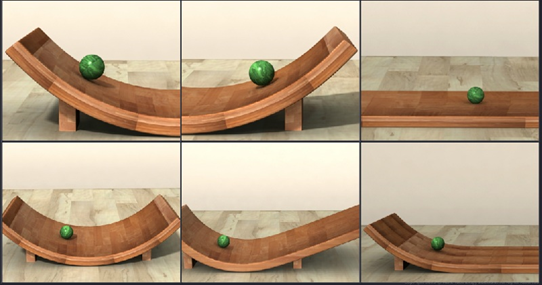Notes On Introduction to Laws of Motion and Inertia - CBSE Class 11 Physics
A force is required to move a stationary body and to stop a moving body. It can be either a contact force or a non-contact force.  According to Aristotle, an external force is required to keep a body in motion. But this is valid only in our world, where frictional forces act on bodies. In the absence of friction, like in space, no external force is required to maintain uniform motion in straight line.  Galileo proved with the help of two experiments that no external force is required to move a body with uniform velocity in the absence of friction.   In the first experiment, a roller rolling down an inclined plane, on its own, accelerates. A roller rolling up an inclined plane, on its own, retards. A roller rolling on a horizontal plane neither accelerates nor retards, i.e., it moves with constant velocity when there is no friction between the roller and the horizontal plane.In the second experiment, when the slope of the two inclined planes is the same, a roller released from rest on one plane rolls down that plane and climbs up the other plane to the same height from which it was released, or a little less. When the slope of the second plane is reduced, the roller climbs up to the same height and travels a longer distance. When the slope of the second plane is made zero, the roller moves infinite distance. This happens only when there is no friction. Actually, the roller comes to rest at some distance due to friction.   Galileo concluded that in either case - the state of rest and the state of uniform linear motion with constant velocity - the net force exerted on a body by the external agencies is zero. Therefore, to maintain uniform velocity, the external force is required only to balance the frictional force.   When the net external force applied on a body is zero, a body at rest remains at rest, and a body moving with uniform velocity in a straight line continues to do so. The tendency of a body at rest to remain at rest or of a body moving with uniform velocity in a straight line to stay in motion with the same uniform velocity, when the net external force is zero, is called inertia.

#### Summary

A force is required to move a stationary body and to stop a moving body. It can be either a contact force or a non-contact force.  According to Aristotle, an external force is required to keep a body in motion. But this is valid only in our world, where frictional forces act on bodies. In the absence of friction, like in space, no external force is required to maintain uniform motion in straight line.  Galileo proved with the help of two experiments that no external force is required to move a body with uniform velocity in the absence of friction.   In the first experiment, a roller rolling down an inclined plane, on its own, accelerates. A roller rolling up an inclined plane, on its own, retards. A roller rolling on a horizontal plane neither accelerates nor retards, i.e., it moves with constant velocity when there is no friction between the roller and the horizontal plane.In the second experiment, when the slope of the two inclined planes is the same, a roller released from rest on one plane rolls down that plane and climbs up the other plane to the same height from which it was released, or a little less. When the slope of the second plane is reduced, the roller climbs up to the same height and travels a longer distance. When the slope of the second plane is made zero, the roller moves infinite distance. This happens only when there is no friction. Actually, the roller comes to rest at some distance due to friction.   Galileo concluded that in either case - the state of rest and the state of uniform linear motion with constant velocity - the net force exerted on a body by the external agencies is zero. Therefore, to maintain uniform velocity, the external force is required only to balance the frictional force.   When the net external force applied on a body is zero, a body at rest remains at rest, and a body moving with uniform velocity in a straight line continues to do so. The tendency of a body at rest to remain at rest or of a body moving with uniform velocity in a straight line to stay in motion with the same uniform velocity, when the net external force is zero, is called inertia.

Previous
Next